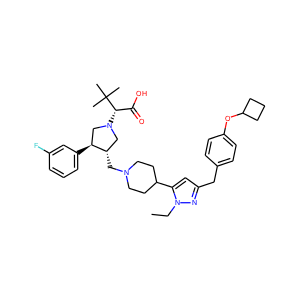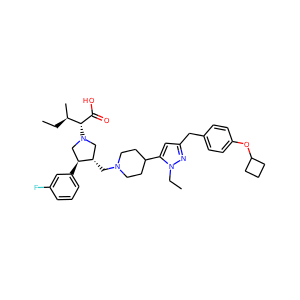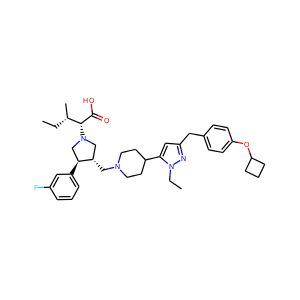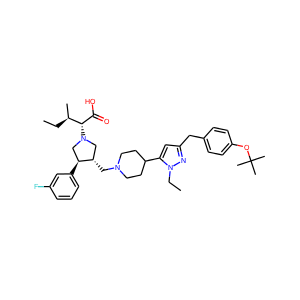## Thursday, August 28, 2014

### MMPA on ChEMBL hERG data using Pandas

The idea here is to try out using Pandas to visualize and work with the output of Jameed Hussain's MMPA code in the IPython notebook. The code is available in the RDKit Contrib dir. Jameed gave a couple tutorials on use of the tools at the 2013 UGM. The notebooks from his tutorials are here and here.
I'll use a ChEMBL hERG dataset. This was somewhat inspired/informed by Paul's work here: https://github.com/pzc/herg_chembl_jcim
This is another post that was too big for blogger. The full post in viewable in the nbviewer here: http://nbviewer.ipython.org/github/greglandrum/rdkit_blog/blob/master/notebooks/ChEMBL%20hERG%20MMPA.ipynb?raw=true.
In :
```from rdkit import Chem,DataStructs
import time,random
from collections import defaultdict
import psycopg2
from rdkit.Chem import Draw,PandasTools,rdMolDescriptors
from rdkit.Chem.Draw import IPythonConsole
from rdkit import rdBase
from __future__ import print_function
import requests
from xml.etree import ElementTree
import pandas as pd
%load_ext sql
print(rdBase.rdkitVersion)
```
```2014.09.1pre

```

# Preparation

Start by finding the hERG data in ChEMBL
In :
```%sql postgresql://localhost/chembl_19 \
select * from chembl_id_lookup where chembl_id = 'CHEMBL240';
```
```1 rows affected.

```
Out:
chembl_id entity_type entity_id status
CHEMBL240 TARGET 165 ACTIVE
In :
```%sql select count(*) from activities join assays using (assay_id) where tid=165;
```
```1 rows affected.

```
Out:
count
14397
Look at all the activity units available.
In :
```%sql select distinct(standard_type) from activities join assays using (assay_id) where tid=165;
```
```27 rows affected.

```
Out:
standard_type
Ratio
Fold change
Ratio IC50
EC25
Imax
EC50
Activity
IC25
IC60
EC10
IP
IC50
QT interval
Time
Ratio Ki
Log IC50
ED50
pIC50
Inflection point
Inhibition
Ki
IC20
V1/2
Potency
INH
pKi
log IC50
In :
```%sql select count(*) from activities join assays using (assay_id) where tid=165 and standard_type='Ki';
```
```1 rows affected.

```
Out:
count
2327

# Create the data set

Pull all hERG assay Ki values where the value is not qualified and the SMILES doesn't include a dot (the MMPA code doesn't get along with dot-separated SMILES).
Reproducibility note: though the queries here are shown against chembl_19, I did the original data export from chembl_18, so the pairs shown may differ somewhat from what you'd get.
In :
```data = %sql select canonical_smiles,molregno,activity_id,standard_value,standard_units from activities \
join assays using (assay_id) \
join compound_structures using (molregno) \
where tid=165 and standard_type='Ki' and standard_value is not null and standard_relation='=' \
and canonical_smiles not like '%.%';
```
```1099 rows affected.

```
Convert to a Pandas DataFrame and write it to a text file
In :
```df = data.DataFrame()
```
In :
```df.to_csv('../data/herg_data.txt',sep=" ",index=False)
```

# Build the matched pairs

Call the MMPA fragmentation program.
This can take a few minutes.
In :
```!python \$RDBASE/Contrib/mmpa/rfrag.py < ../data/herg_data.txt > ../data/herg_fragmented.txt
```
```[03:43:47] SMILES Parse Error: syntax error for input: canonical_smiles
Can't generate mol for: canonical_smiles

```
Now generate the MMPs that differ by less than 10% of the molecule. Generate symmetrically so that we can detect tforms in both directions.
In :
```!python \$RDBASE/Contrib/mmpa/indexing.py -s -r 0.1 < ../data/herg_fragmented.txt > ../data/mmps_default.txt
```
Read those into a Pandas data frame and look at some of the data.
In :
```mmps = pd.read_csv('../data/mmps_default.txt',header=None,names=('smiles1','smiles2','molregno1','molregno2','tform','core'))
```
In :
```mmps[mmps.molregno1==290813]
```
Out:
smiles1 smiles2 molregno1 molregno2 tform core
0 CCn1nc(Cc2ccc(OC3CCC3)cc2)cc1C4CCN(C[C@H]5CN(C[C@@H]5c6cccc(F)c6)[C@@H](C(=O)O)C(C)(C)C)CC4 CC[C@@H](C)[C@@H](N1C[C@H](CN2CCC(CC2)c3cc(Cc4ccc(OC5CCC5)cc4)nn3CC)[C@H](C1)c6cccc(F)c6)C(=O)O 290813 290814 [*:1]C(C)(C)C>>[*:1][C@H](C)CC [*:1][C@H](C(=O)O)N1C[C@H](CN2CCC(c3cc(Cc4ccc(OC5CCC5)cc4)nn3CC)CC2)[C@@H](c2cccc(F)c2)C1
2 CCn1nc(Cc2ccc(OC3CCC3)cc2)cc1C4CCN(C[C@H]5CN(C[C@@H]5c6cccc(F)c6)[C@@H](C(=O)O)C(C)(C)C)CC4 CC[C@H](C)[C@@H](N1C[C@H](CN2CCC(CC2)c3cc(Cc4ccc(OC5CCC5)cc4)nn3CC)[C@H](C1)c6cccc(F)c6)C(=O)O 290813 290815 [*:1]C(C)(C)C>>[*:1][C@@H](C)CC [*:1][C@H](C(=O)O)N1C[C@H](CN2CCC(c3cc(Cc4ccc(OC5CCC5)cc4)nn3CC)CC2)[C@@H](c2cccc(F)c2)C1
92 CCn1nc(Cc2ccc(OC3CCC3)cc2)cc1C4CCN(C[C@H]5CN(C[C@@H]5c6cccc(F)c6)[C@@H](C(=O)O)C(C)(C)C)CC4 CC[C@@H](C)[C@@H](N1C[C@H](CN2CCC(CC2)c3cc(Cc4ccc(OC5CCC5)cc4)nn3CC)[C@H](C1)c6cccc(F)c6)C(=O)O 290813 290814 [*:1]C([*:2])([*:3])C>>[*:1]CC([*:2])[*:3] [*:3]C.[*:1]C.[*:2][C@H](C(=O)O)N1C[C@H](CN2CCC(c3cc(Cc4ccc(OC5CCC5)cc4)nn3CC)CC2)[C@@H](c2cccc(F)c2)C1
94 CCn1nc(Cc2ccc(OC3CCC3)cc2)cc1C4CCN(C[C@H]5CN(C[C@@H]5c6cccc(F)c6)[C@@H](C(=O)O)C(C)(C)C)CC4 CC[C@H](C)[C@@H](N1C[C@H](CN2CCC(CC2)c3cc(Cc4ccc(OC5CCC5)cc4)nn3CC)[C@H](C1)c6cccc(F)c6)C(=O)O 290813 290815 [*:1]C([*:2])([*:3])C>>[*:1]CC([*:2])[*:3] [*:3]C.[*:1]C.[*:2][C@H](C(=O)O)N1C[C@H](CN2CCC(c3cc(Cc4ccc(OC5CCC5)cc4)nn3CC)CC2)[C@@H](c2cccc(F)c2)C1
1368 CCn1nc(Cc2ccc(OC3CCC3)cc2)cc1C4CCN(C[C@H]5CN(C[C@@H]5c6cccc(F)c6)[C@@H](C(=O)O)C(C)(C)C)CC4 CC[C@@H](C)[C@@H](N1C[C@H](CN2CCC(CC2)c3cc(Cc4ccc(OC5CCC5)cc4)nn3CC)[C@H](C1)c6cccc(F)c6)C(=O)O 290813 290814 [*:1]C([*:2])(C)C>>[*:1]C([*:2])CC [*:1]C.[*:2][C@H](C(=O)O)N1C[C@H](CN2CCC(c3cc(Cc4ccc(OC5CCC5)cc4)nn3CC)CC2)[C@@H](c2cccc(F)c2)C1
1370 CCn1nc(Cc2ccc(OC3CCC3)cc2)cc1C4CCN(C[C@H]5CN(C[C@@H]5c6cccc(F)c6)[C@@H](C(=O)O)C(C)(C)C)CC4 CC[C@@H](C)[C@@H](N1C[C@H](CN2CCC(CC2)c3cc(Cc4ccc(OC5CCC5)cc4)nn3CC)[C@H](C1)c6cccc(F)c6)C(=O)O 290813 290814 [*:1]C([*:2])(C)C>>[*:1]C[C@H]([*:2])C [*:1]C.[*:2][C@H](C(=O)O)N1C[C@H](CN2CCC(c3cc(Cc4ccc(OC5CCC5)cc4)nn3CC)CC2)[C@@H](c2cccc(F)c2)C1
1372 CCn1nc(Cc2ccc(OC3CCC3)cc2)cc1C4CCN(C[C@H]5CN(C[C@@H]5c6cccc(F)c6)[C@@H](C(=O)O)C(C)(C)C)CC4 CC[C@H](C)[C@@H](N1C[C@H](CN2CCC(CC2)c3cc(Cc4ccc(OC5CCC5)cc4)nn3CC)[C@H](C1)c6cccc(F)c6)C(=O)O 290813 290815 [*:1]C([*:2])(C)C>>[*:1]C[C@@H]([*:2])C [*:1]C.[*:2][C@H](C(=O)O)N1C[C@H](CN2CCC(c3cc(Cc4ccc(OC5CCC5)cc4)nn3CC)CC2)[C@@H](c2cccc(F)c2)C1
1374 CCn1nc(Cc2ccc(OC3CCC3)cc2)cc1C4CCN(C[C@H]5CN(C[C@@H]5c6cccc(F)c6)[C@@H](C(=O)O)C(C)(C)C)CC4 CC[C@H](C)[C@@H](N1C[C@H](CN2CCC(CC2)c3cc(Cc4ccc(OC5CCC5)cc4)nn3CC)[C@H](C1)c6cccc(F)c6)C(=O)O 290813 290815 [*:1]C([*:2])(C)C>>[*:1]C([*:2])CC [*:1]C.[*:2][C@H](C(=O)O)N1C[C@H](CN2CCC(c3cc(Cc4ccc(OC5CCC5)cc4)nn3CC)CC2)[C@@H](c2cccc(F)c2)C1
1470 CCn1nc(Cc2ccc(OC3CCC3)cc2)cc1C4CCN(C[C@H]5CN(C[C@@H]5c6cccc(F)c6)[C@@H](C(=O)O)C(C)(C)C)CC4 CCn1nc(Cc2ccc(OC(C)C)cc2)cc1C3CCN(C[C@H]4CN(C[C@@H]4c5cccc(F)c5)[C@@H](C(=O)O)C(C)(C)C)CC3 290813 290921 [*:1]C1CCC1>>[*:1]C(C)C [*:1]Oc1ccc(Cc2cc(C3CCN(C[C@H]4CN([C@@H](C(=O)O)C(C)(C)C)C[C@@H]4c4cccc(F)c4)CC3)n(CC)n2)cc1
1472 CCn1nc(Cc2ccc(OC3CCC3)cc2)cc1C4CCN(C[C@H]5CN(C[C@@H]5c6cccc(F)c6)[C@@H](C(=O)O)C(C)(C)C)CC4 CCn1nc(Cc2ccc(OC(F)(F)F)cc2)cc1C3CCN(C[C@H]4CN(C[C@@H]4c5cccc(F)c5)[C@@H](C(=O)O)C(C)(C)C)CC3 290813 292218 [*:1]C1CCC1>>[*:1]C(F)(F)F [*:1]Oc1ccc(Cc2cc(C3CCN(C[C@H]4CN([C@@H](C(=O)O)C(C)(C)C)C[C@@H]4c4cccc(F)c4)CC3)n(CC)n2)cc1
1504 CCn1nc(Cc2ccc(OC3CCC3)cc2)cc1C4CCN(C[C@H]5CN(C[C@@H]5c6cccc(F)c6)[C@@H](C(=O)O)C(C)(C)C)CC4 CC[C@@H](C)[C@@H](N1C[C@H](CN2CCC(CC2)c3cc(Cc4ccc(OC5CCC5)cc4)nn3CC)[C@H](C1)c6cccc(F)c6)C(=O)O 290813 290814 [*:1]C([*:2])C([*:3])(C)C>>[*:1]C([*:2])[C@@H]([*:3])CC [*:3]C.[*:1]C(=O)O.[*:2]N1C[C@H](CN2CCC(c3cc(Cc4ccc(OC5CCC5)cc4)nn3CC)CC2)[C@@H](c2cccc(F)c2)C1
1506 CCn1nc(Cc2ccc(OC3CCC3)cc2)cc1C4CCN(C[C@H]5CN(C[C@@H]5c6cccc(F)c6)[C@@H](C(=O)O)C(C)(C)C)CC4 CC[C@@H](C)[C@@H](N1C[C@H](CN2CCC(CC2)c3cc(Cc4ccc(OC5CCC5)cc4)nn3CC)[C@H](C1)c6cccc(F)c6)C(=O)O 290813 290814 [*:1]C([*:2])C([*:3])(C)C>>[*:3]C[C@@H](C)C([*:1])[*:2] [*:3]C.[*:1]C(=O)O.[*:2]N1C[C@H](CN2CCC(c3cc(Cc4ccc(OC5CCC5)cc4)nn3CC)CC2)[C@@H](c2cccc(F)c2)C1
1508 CCn1nc(Cc2ccc(OC3CCC3)cc2)cc1C4CCN(C[C@H]5CN(C[C@@H]5c6cccc(F)c6)[C@@H](C(=O)O)C(C)(C)C)CC4 CC[C@H](C)[C@@H](N1C[C@H](CN2CCC(CC2)c3cc(Cc4ccc(OC5CCC5)cc4)nn3CC)[C@H](C1)c6cccc(F)c6)C(=O)O 290813 290815 [*:1]C([*:2])C([*:3])(C)C>>[*:1]C([*:2])[C@H]([*:3])CC [*:3]C.[*:1]C(=O)O.[*:2]N1C[C@H](CN2CCC(c3cc(Cc4ccc(OC5CCC5)cc4)nn3CC)CC2)[C@@H](c2cccc(F)c2)C1
1510 CCn1nc(Cc2ccc(OC3CCC3)cc2)cc1C4CCN(C[C@H]5CN(C[C@@H]5c6cccc(F)c6)[C@@H](C(=O)O)C(C)(C)C)CC4 CC[C@H](C)[C@@H](N1C[C@H](CN2CCC(CC2)c3cc(Cc4ccc(OC5CCC5)cc4)nn3CC)[C@H](C1)c6cccc(F)c6)C(=O)O 290813 290815 [*:1]C([*:2])C([*:3])(C)C>>[*:3]C[C@H](C)C([*:1])[*:2] [*:3]C.[*:1]C(=O)O.[*:2]N1C[C@H](CN2CCC(c3cc(Cc4ccc(OC5CCC5)cc4)nn3CC)CC2)[C@@H](c2cccc(F)c2)C1
There are dupes... drop them:
In :
```mmps=mmps.drop_duplicates(subset=("molregno1","molregno2"))
```
Add a couple molecule columns and remove the SMILES columns:
In :
```PandasTools.AddMoleculeColumnToFrame(mmps,'smiles1','mol1')
PandasTools.AddMoleculeColumnToFrame(mmps,'smiles2','mol2')
mmps = mmps[['mol1','mol2','molregno1','molregno2','tform','core']]
mmps.head()
```
Out:
mol1 mol2 molregno1 molregno2 tform core
0290813 290814 [*:1]C(C)(C)C>>[*:1][C@H](C)CC [*:1][C@H](C(=O)O)N1C[C@H](CN2CCC(c3cc(Cc4ccc(OC5CCC5)cc4)nn3CC)CC2)[C@@H](c2cccc(F)c2)C1
1290814 290813 [*:1][C@H](C)CC>>[*:1]C(C)(C)C [*:1][C@H](C(=O)O)N1C[C@H](CN2CCC(c3cc(Cc4ccc(OC5CCC5)cc4)nn3CC)CC2)[C@@H](c2cccc(F)c2)C1
2290813 290815 [*:1]C(C)(C)C>>[*:1][C@@H](C)CC [*:1][C@H](C(=O)O)N1C[C@H](CN2CCC(c3cc(Cc4ccc(OC5CCC5)cc4)nn3CC)CC2)[C@@H](c2cccc(F)c2)C1
3290815 290813 [*:1][C@@H](C)CC>>[*:1]C(C)(C)C [*:1][C@H](C(=O)O)N1C[C@H](CN2CCC(c3cc(Cc4ccc(OC5CCC5)cc4)nn3CC)CC2)[C@@H](c2cccc(F)c2)C1
4290814 290815 [*:1][C@H](C)CC>>[*:1][C@@H](C)CC [*:1][C@H](C(=O)O)N1C[C@H](CN2CCC(c3cc(Cc4ccc(OC5CCC5)cc4)nn3CC)CC2)[C@@H](c2cccc(F)c2)C1
Now join back on the original data so that we have activities in the table again. Pandas makes this easy.
In :
```t1=df[['molregno','standard_value']]
mmpdds = mmps.merge(t1,left_on='molregno1',right_on='molregno',suffixes=("_1","_2")).\
merge(t1,left_on='molregno2',right_on='molregno',suffixes=("_1","_2"))
mmpdds.head()
```
Out:
mol1 mol2 molregno1 molregno2 tform core molregno_1 standard_value_1 molregno_2 standard_value_2
0290813 290814 [*:1]C(C)(C)C>>[*:1][C@H](C)CC [*:1][C@H](C(=O)O)N1C[C@H](CN2CCC(c3cc(Cc4ccc(OC5CCC5)cc4)nn3CC)CC2)[C@@H](c2cccc(F)c2)C1 290813 3500 290814 3400
1290815 290814 [*:1][C@@H](C)CC>>[*:1][C@H](C)CC [*:1][C@H](C(=O)O)N1C[C@H](CN2CCC(c3cc(Cc4ccc(OC5CCC5)cc4)nn3CC)CC2)[C@@H](c2cccc(F)c2)C1 290815 5700 290814 3400
2290879 290814 [*:1]C(C)(C)C>>[*:1]C1CCC1 [*:1]Oc1ccc(Cc2cc(C3CCN(C[C@H]4CN([C@@H](C(=O)O)[C@H](C)CC)C[C@@H]4c4cccc(F)c4)CC3)n(CC)n2)cc1 290879 5600 290814 3400
3290813 290815 [*:1]C(C)(C)C>>[*:1][C@@H](C)CC [*:1][C@H](C(=O)O)N1C[C@H](CN2CCC(c3cc(Cc4ccc(OC5CCC5)cc4)nn3CC)CC2)[C@@H](c2cccc(F)c2)C1 290813 3500 290815 5700
4290814 290815 [*:1][C@H](C)CC>>[*:1][C@@H](C)CC [*:1][C@H](C(=O)O)N1C[C@H](CN2CCC(c3cc(Cc4ccc(OC5CCC5)cc4)nn3CC)CC2)[C@@H](c2cccc(F)c2)C1 290814 3400 290815 5700
Calculate pKi values and the difference between them:
In :
```import math
mmpdds['pKi_1']=mmpdds.apply(lambda row:-1*math.log10(float(row['standard_value_1'])*1e-9),axis=1)
mmpdds['pKi_2']=mmpdds.apply(lambda row:-1*math.log10(float(row['standard_value_2'])*1e-9),axis=1)
mmpdds['delta']=mmpdds['pKi_2']-mmpdds['pKi_1']
```
And, remove some extra columns:
In :
```mmpdds=mmpdds[['mol1','mol2','molregno1','molregno2','pKi_1','pKi_2','delta','tform','core']]
mmpdds.head()
```
Out:
mol1 mol2 molregno1 molregno2 pKi_1 pKi_2 delta tform core
0290813 290814 5.455932 5.468521 0.012589 [*:1]C(C)(C)C>>[*:1][C@H](C)CC [*:1][C@H](C(=O)O)N1C[C@H](CN2CCC(c3cc(Cc4ccc(OC5CCC5)cc4)nn3CC)CC2)[C@@H](c2cccc(F)c2)C1
1290815 290814 5.244125 5.468521 0.224396 [*:1][C@@H](C)CC>>[*:1][C@H](C)CC [*:1][C@H](C(=O)O)N1C[C@H](CN2CCC(c3cc(Cc4ccc(OC5CCC5)cc4)nn3CC)CC2)[C@@H](c2cccc(F)c2)C1
2290879 290814 5.251812 5.468521 0.216709 [*:1]C(C)(C)C>>[*:1]C1CCC1 [*:1]Oc1ccc(Cc2cc(C3CCN(C[C@H]4CN([C@@H](C(=O)O)[C@H](C)CC)C[C@@H]4c4cccc(F)c4)CC3)n(CC)n2)cc1
3290813 290815 5.455932 5.244125 -0.211807 [*:1]C(C)(C)C>>[*:1][C@@H](C)CC [*:1][C@H](C(=O)O)N1C[C@H](CN2CCC(c3cc(Cc4ccc(OC5CCC5)cc4)nn3CC)CC2)[C@@H](c2cccc(F)c2)C1
4290814 290815 5.468521 5.244125 -0.224396 [*:1][C@H](C)CC>>[*:1][C@@H](C)CC [*:1][C@H](C(=O)O)N1C[C@H](CN2CCC(c3cc(Cc4ccc(OC5CCC5)cc4)nn3CC)CC2)[C@@H](c2cccc(F)c2)C1

# Analysis

Let's start by grouping related transforms together and seeing how often they occur:
Unfortunately, this is about where blogger started choking on the code. You can view the full post in the nbviewer: http://nbviewer.ipython.org/github/greglandrum/rdkit_blog/blob/master/notebooks/ChEMBL%20hERG%20MMPA.ipynb?raw=true.